# Dirac algebra

(diff) ← Older revision | Latest revision (diff) | Newer revision → (diff)

The Dirac algebra arises from Dirac's solution [a3] to the relativistic electron equation: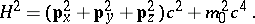Dirac found hypercomplex elements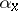,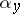,and(cf. also Hypercomplex number) such that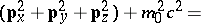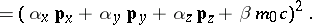The Hamiltonian is given, after the usual substitutions for the linear momentum components, by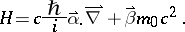The time-dependent equation is known as Dirac's equation: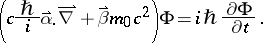The elements,,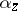andsatisfy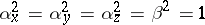and the anti-commutativity relations: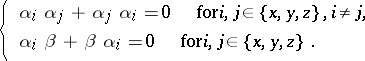The Dirac representation of the matricesandis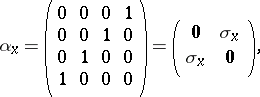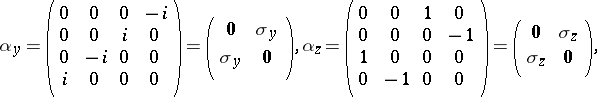where,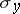and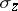are the Pauli spin matrices (cf. also Pauli matrices; Dirac matrices). This choice is not unique; pre-multiplying by any unitary matrixand post-multiplying by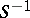will produce a new set of matrices satisfying the conditions. The defining relations are often expressed more abstractly by the Dirac gamma matrices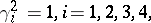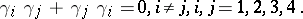The Dirac algebra is the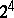-dimensional complex Clifford algebra generated by the gamma matrices under the usual matrix operations and is isomorphic to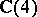, the ring of four-by-four matrices over the complex numbers. The use of the complex numbers as scalars apparently is motivated by the fact that complex numbers are use to express solutions to the Schrödinger wave equations (cf. also Schrödinger equation).

Two other "Dirac algebras" commonly appear in the literature [a4]; in each the relation among the squares of the generating elements, the metric, has been modified and the scalar field is the real numbers. Upon complexification, both become the algebra. The modified metrics are the metrics of Minkowski space-time (cf. also Minkowski space) and more easily illustrate the physics or the geometry.

In one case (see, for example [a2]) the metric is given by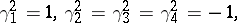the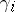generate a-dimensional real Clifford algebra that is isomorphic to, the ring of two-by-two matrices over the real quaternion division ring. Every Clifford algebraadmits a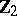-grading,such that for all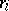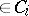and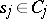,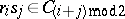. The subspace, spanned by the identity element and all products of an even number, is, in the present case, a subalgebra isomorphic to to the-dimensional Pauli algebra.

The Majorana representation is the-dimensional real Clifford algebra with metric (as in [a1])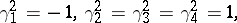that is isomorphic to. Here, the elements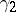,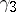and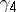generate a subalgebra isomorphic to to the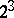-dimensional Pauli algebra.

How to Cite This Entry:
Dirac algebra. Encyclopedia of Mathematics. URL: http://encyclopediaofmath.org/index.php?title=Dirac_algebra&oldid=13746
This article was adapted from an original article by G.P. Wene (originator), which appeared in Encyclopedia of Mathematics - ISBN 1402006098. See original article